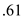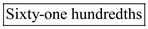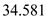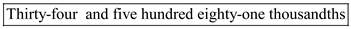# Quiz 3: Decimals

Business

The number to be written in the word form isFirst write the number sixty-one. Because the last digit, 1, is in the hundredths place the decimal would be written as: Sixty-one hundredths Thus, the solution is.

The answer is decimals. Decimals are used extensively in business applications.

The number to be written in the word form isStart by writing the whole number thirty-four. Then write and for the decimal point. Write the number five hundred eighty-one. Because the last digit, 1, is in the thousandths place the decimal would be written as: Thirty-four and five hundred eighty-one thousandths. Thus, the solution is.

There is no answer for this question

There is no answer for this question

There is no answer for this question

There is no answer for this question